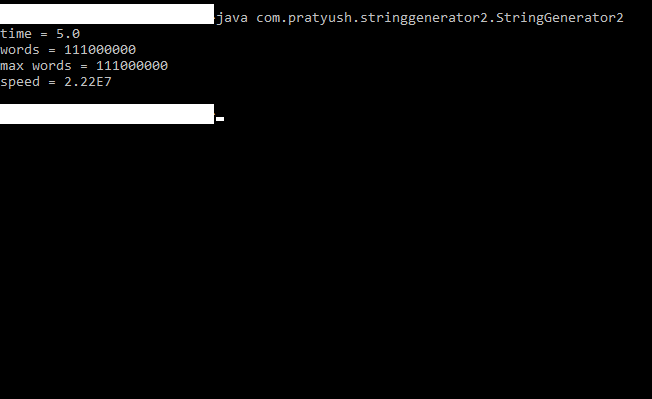# How to generate combinations from a set of characters

In this post, I shall share a code sample in Java that generates string combinations of characters from a given set of characters over a specified range of lengths of such string combinations. I hope I am not boring you all with this topic,since it’s my third such code sample to this effect. The other two samples can be found here and here.

Note that this is my fastest such code yet. It maxes out the cores of a multi-core CPU on execution. Also note that I have no systematic algorithm for this one! Does that sound weird?

There is no exponentiation involved in this sample as in the one here. We simply map elements in an integer array to characters in a character array and then convert that character array to a string when we want to do something with it.

We manipulate indices in the integer array by incrementation and decrementation, that’s it. The code uses the concurrency APIs of Java and is fully parallelized. It achieved about twenty two million iterations per second during my tests.

Finally, let me say further that I take no responsibility for what is done with this code.

```package com.pratyush.stringgenerator2;

import java.util.concurrent.*;

class StringGenerator2 implements Runnable
{
private static char[] dictionary = {'a', 'b', 'c', 'd', 'e', 'f', 'g', 'h', 'i', 'j'};

static Object locker;

static  ExecutorService es;

static int attempts = 0;

private static String getStringFromIndices(int[] indices, char[] str)
{

for(int z = 0; z < str.length; z++)
{
str[z]=dictionary[indices[z]];
}

return new String(str);

}

private static long getMaxAttempts(int startLen, int stopLen)
{
long myMaxAttempts = 0;

for(int c = startLen; c <= stopLen; c++)
{
myMaxAttempts += Math.pow(dictionary.length, c);
}

return myMaxAttempts;
}

public void run()
{

long start = System.currentTimeMillis();

generate(6, 8);

long end = System.currentTimeMillis();

double secs = (end - start)/1000;

System.out.println("time = "+secs);

System.out.println("words = "+attempts);

System.out.println("max words = "+ getMaxAttempts(6,8));

double speed = attempts / secs;

System.out.println("speed = "+speed);

synchronized(locker)
{

es.shutdownNow();

locker.notify();
}

}

public static void generate(int startLen, int stopLen)
{
int LEN = dictionary.length;

attempts = 0;

String strCombo = "";

for(int currLen = startLen; currLen<= stopLen; currLen++)
{
char[] str = new char[currLen];

int[] indexes = new int[currLen];

for(int f = 0; f < indexes.length; f++)
{
indexes[f] = 0;
}

for(int j = 0; j < LEN; j++ )
{
indexes = j;

if(currLen == 1)
{

strCombo = getStringFromIndices(indexes, str);

/***generate string here and do whatever you like***/

attempts++;
}
else
{
while(indexes<LEN)
{
int i = 1;

for(i = 1; i < currLen; i++)
{
if(indexes[i] == LEN)
{
break;
}

if(i == currLen - 1)
{

strCombo = getStringFromIndices(indexes, str);

/***generate string here and do whatever you like***/

indexes[i]++;
attempts++;
}
}

for(int k = 2;k<currLen;k++)
{
if(indexes[k] == LEN)
{
indexes[k-1]++;

for(int l = k; l<currLen; l++)
{
indexes[l] = 0;
}
}
}
}

for(int m = 1; m < currLen; m++)
{
indexes[m] = 0;
}
}
}
}

}

public static void main(String[] args)
{

locker = new Object();

es.execute(new StringGenerator2());

try{
synchronized(locker)
{
locker.wait();
}
}
catch(InterruptedException ie)
{
ie.printStackTrace();
}

}
}```

Code execution after compilation is shown below: